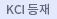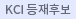#다국어 입력

http://chineseinput.net/에서 pinyin(병음)방식으로 중국어를 변환할 수 있습니다.

변환된 중국어를 복사하여 사용하시면 됩니다.

예시)
• 中文 을 입력하시려면 zhongwen을 입력하시고 space를누르시면됩니다.
• 北京 을 입력하시려면 beijing을 입력하시고 space를 누르시면 됩니다.
닫기확인

검색결과 좁혀 보기

• 좁혀본 항목 보기순서

• 원문유무
• 음성지원유무
• 원문제공처
• 등재정보
• 학술지명
• 주제분류
• 발행연도
• 작성언어
• 저자

오늘 본 자료

• 오늘 본 자료가 없습니다.
더보기
• 무료
• 기관 내 무료
• 유료
•RF 마그네트론 스퍼터링법으로 제작된 ITO 박막의 공정압력 변화에 따른 특성

The transparent electrode properties of ITO films deposited by RF magnetron sputtering with process pressure were investigated. The ITO thin films was deposited on a glass substrate using a target with 3in diameter sintered at a ratio of $In_2O_3$ : $SnO_2$ (9 : 1). 200-nm-thick ITO thin films were manufactured by various process pressures ($2.0{\times}10^{-2}$, $7.0{\times}10^{-3}$ and $2.0{\times}10^{-3}$ Torr). The optical transmittance and resistivity of the deposited ITO thin films showed a relatively satisfactory result under $10^{-2}$ Torr. For high process pressure, the optical transmittance was below 80%, while for low process pressure, the optical transmittance was above 85%. As a result of of mobility, resistivity and carrier concentration by Hall measurement, we obtained satisfactory properties to apply into a transparent conducting thin film.

•심플렉스 기법의 복잡성에 관한 연구

We show that the complexity of Simplex Method for Linear Programming problem is equivalent to the complexity of finding just an adjacent basic feasible solution if exists. Therefore a simplex type method which resolves degeneracy in polynomial time with respect to the size of the given linear programming problem can solve the general linear programming problem in polynomial steps.

•RF 마그네트론 스퍼터링법으로 제작된 Ga-doped ZnO 박막의 공정압력에 따른 전기적, 광학적 특성

투명전도산화막인 Ga-도핑된 ZnO (GZO) 박막을 RF 마그네트론 스퍼터링 증착법을 이용하여 증착하고 전기적, 광학적 특성을 연구하였다. 증착변수로 공정압력에 변수를 주었으며 공정 압력 변화에 따라 전기적 특성과 광학적 특성이 달라짐을 확인할 수 있었다. 모든 박막은 공정압력에 상관없이 c-축(002) 방향성을 나타냈다. 증착된 GZO 박막의 전기저항성은 $8.68{\times}10^{-3}{\Omega}{\cdot}cm\sim2.18{\times}10^{-3}{\Omega}{\cdot}cm$이었고, 모든 가시광 영역에서 90% 이상의 평균 투과율을 보였다. 공정압력에 따라 상온에서 증착된 GZO 박막은 우수한 낮은 저항성과 높은 투과율을 나타내었고, 평판디스플레이와 태양전지의 투명전극으로 응용되기에 적합한 특성을 지닌 것을 확인 할 수 있었다. Ga-doped ZnO (GZO) thin films for application as transparent conducting oxide film were deposited on the glass substrate by using rf-magnetron sputtering system. The effects of working pressure on electrical and optical characteristics of GZO films were investigated. Regardless of the working pressure, all films were oriented along with the c-axis, perpendicular to the substrate. The electrical resistivity was about $8.68{\times}10^{-3}{\Omega}{\cdot}cm\sim2.18{\times}10^{-3}{\Omega}{\cdot}cm$ and the average transmittance of all films including substrates was over 90% in the visible range. The good transparents and conducting properties were obtained due to controle the working pressure. The obtained results have acceptable for application as transparent conductive electrodes in LCDs and solar cells.

•다변환 심플렉스 기법과 이의 효율성

A multi-interchange simplex method is presented. This method tries to cut almost half of the set of convex combinations which generate all decreasing feasible directions. Analysis of this method indicates high possibility of the existence of polynomial-time simplex-type methods.